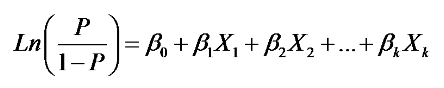# Modelling Probability of Default Using Logistic Regression

While building credit risk models, one of the most important activities performed by banks is to predict the probability of default. Default is the event that a loan borrower will default on his payment obligation during the duration of the loan. The probability of default (PD) is the likelihood of default, that is, the likelihood that the borrower will default on his obligations during the given time period.

When you look at credit scores, such as FICO for consumers, they typically imply a certain probability of default. For example, the FICO score ranges from 300 to 850 with a score of 850 implying the lowest risk of default. This is an important factor considered by lenders while approving or disapproving your loan.

The analysts at banks use various models to model the probability of default such as Logistic model, Probit model, and Neural networks. In this article, we will look at how logistic regression models can be used to create a model to predict the probability of default.

### What is Logistic Regression?

Logistic regression aims to model the probability of an event occurring depending on the values of independent variables.

These independent variables are the various categorical or numerical information available to us regarding the loan, and these variables can help us model the probability of the event (in our case, the probability of default). These variables are also called predictor variables.

Some examples of these predictor variables are provided below:

1. Personal details: Personal details of the borrower such as age, employment status, profession, income, residential status, and number of dependents.
2. Credit history: Length of credit history, number and value of past loans, number and value of past delinquent loans.
3. Behavioral data: Spending pattern, repayment patterns.

All these variables can be used as predictor variables to predict the probability of default. So, using logistic regression, we model the probability of default using other independent variables as described above.

The logistic regression model seeks to estimate that an event (default) will occur for a randomly selected observation versus the probability that the event does not occur. Suppose we have data for 1000 loans along with all the predictor variables and also whether the borrower defaulted on it or not. Here the probability of default is referred to as the response variable or the dependent variable. The default itself is a binary variable, that is, its value will be either 0 or 1 (0 is no default, and 1 is default).

In logistic regression, the dependent variable is binary, i.e. it only contains data marked as 1 (Default) or 0 (No default).

We can say that logistic regression is a classification algorithm used to predict a binary outcome (1 / 0, Default / No Default) given a set of independent variables. It is a special case of linear regression when the outcome variable is categorical. It predicts the probability of occurrence of a default by fitting data to a logit function.

A link function is simply a function of the mean of the response variable Y that we use as the response instead of Y itself. In our example, Y represents default.

All that means is when Y is categorical, we use the logit of Y as the response in our regression equation instead of just Y:The logit function is the natural log of the odds that Y equals one of the categories.  For mathematical simplicity, we’re going to assume Y has only two categories and code them as 0 and 1.

The logit function is the inverse of the logistic transform. When the function's variable represents a probability p, the logit function gives the log-odds, or the logarithm of the odds p/(1 − p). The log-odds score is typically the basis of the credit score used by banks and credit bureaus to rank people.

P is defined as the probability that Y=1 (Representing Default).  So for example, those Xs could be specific risk factors, like age, income, employment status, credit history, and P would be the probability that a borrower defaults. B0 is an intercept and ( B1...Bk) is a vector of coefficients, one for each predictor variable.

# R Programming Bundle: 25% OFF

Get our R Programming - Data Science for Finance Bundle for just $29$39.
Get it now for just \$29